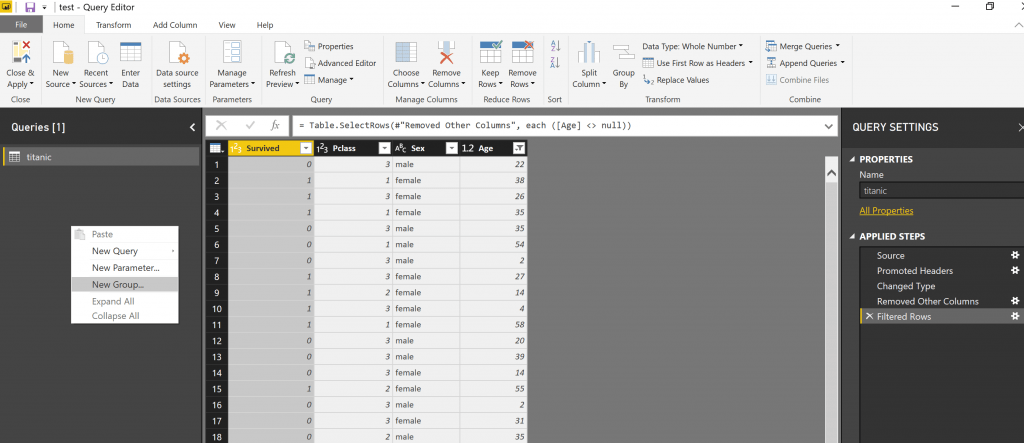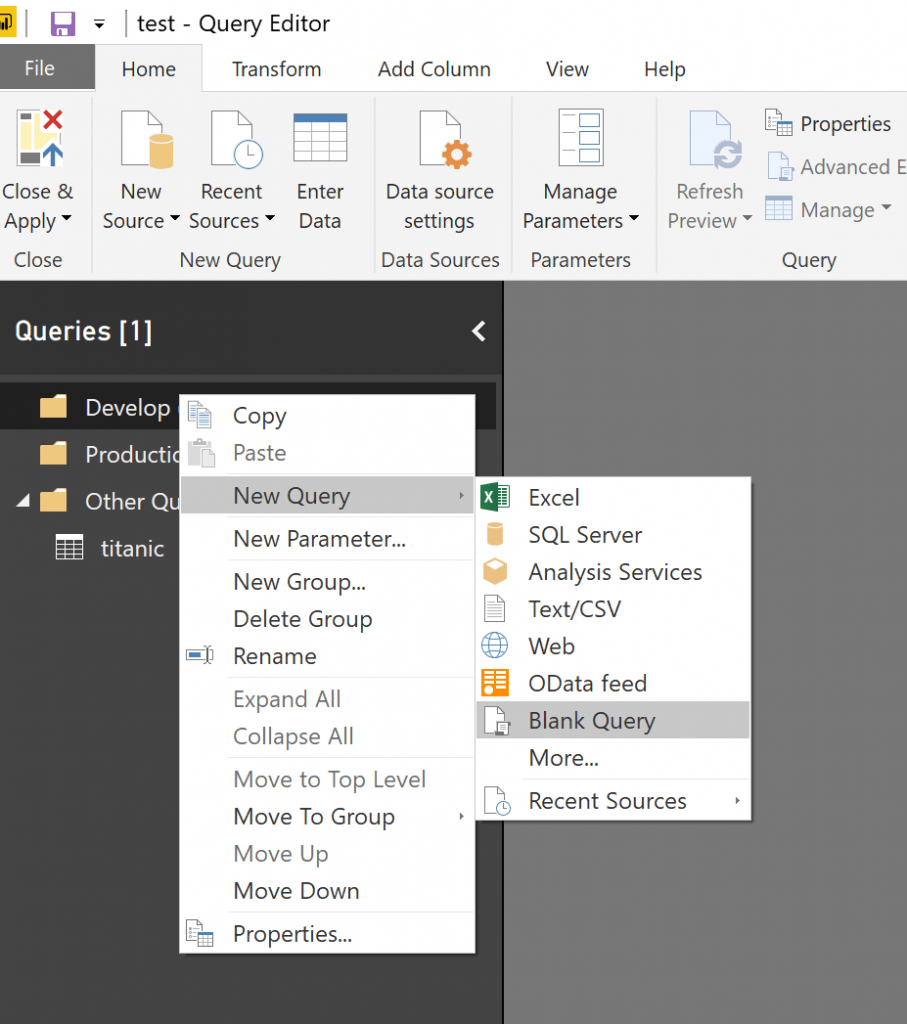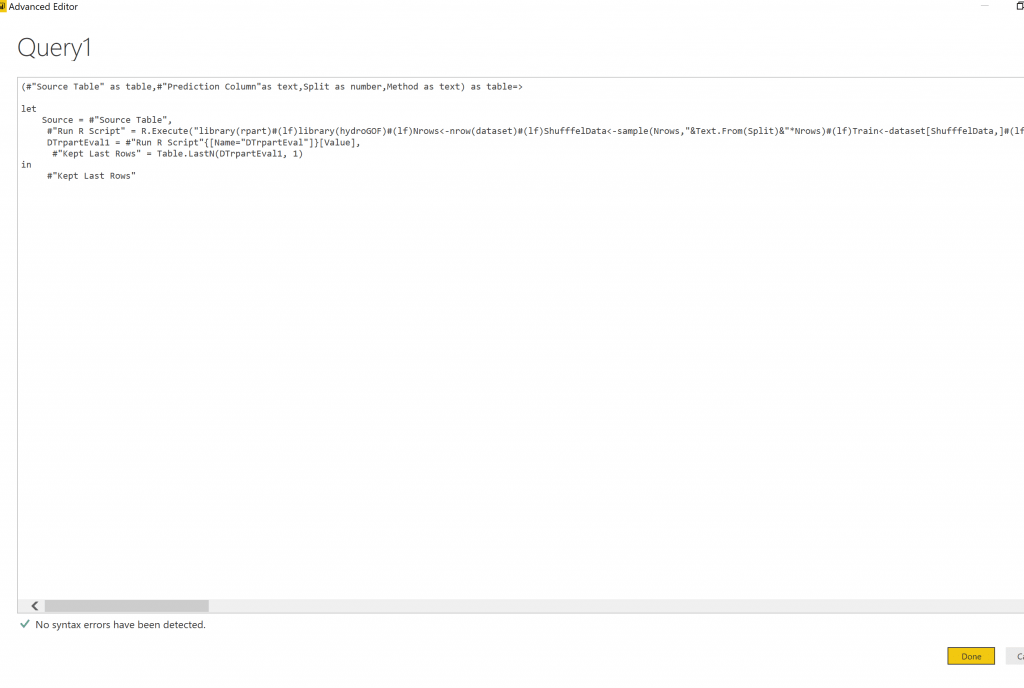# Creating Machine learning Development and Production Environment in Power BI-Part2In the last post, I have explained how we can create a function with the aim of parametrising the machine learning process. In this post, I am going to explain how we able to create a Development and Production environment.### Create Different Environment in Power Query

to create a new environment, In power query, I created two main groups one for “Develop” and the other one for “Production”. To create a different group, I just right-click in the Query window, then create two separate group.## Develop (Test and Train)

First, I create a function to train a model and get the result of the evaluation. In this post, I will show how I leverage “rpart” a decision tree algorithm and “knn” both for classification.

First, I write some R codes to train models and also to evaluate the algorithm.

for “rpart” I have :

Train model for rpart :

```library(rpart)
library(hydroGOF)
Nrows<-nrow(dataset)
ShufffelData<-sample(Nrows,0.8*Nrows)
Train<-dataset[ShufffelData,]
Test<-dataset[-ShufffelData,]
DT <- rpart(Survived~., data = Train, method = "class")
predictions <- predict(DT, Test)
Pred<-data.frame(Test\$Survived)
names(Pred)<-paste("actual")
i=1   for(i in 1:nrow(Test))
if(predictions[i,1]>=predictions[i,2]) {  Pred[i,2]<-"0"} else  {  Pred[i,2]<-"1")}
names(Pred)<-paste("prediction")
```

Test Model

```cm <-as.matrix(table(Actual = Pred\$actual, Predicted =Pred\$prediction ))
sumtest<-sum(cm)
NrowTest<-nrow(cm)
diag <- diag(cm)
rowsums = apply(cm, 1, sum)
colsums = apply(cm, 2, sum)
p = rowsums / sumtest
q = colsums / sumtest
accuracy = sum(diag) / sumtest
precision = diag / colsums
recall = diag / rowsums
f1 = 2 * precision * recall / (precision + recall)
```

Then I follow the steps in the last post, to create a function and pass the parameters such as “dataset” for training, split percentage, the column name for prediction.

the M code for having a function with parameters in Power query to train and show the test result of rpart algorithm would be as below:

```(#"Source Table" as table,#"Prediction Column"as text,Split as number,Method as text) as table=>

let
Source = #"Source Table",
#"Run R Script" = R.Execute("library(rpart)#(lf)library(hydroGOF)#(lf)Nrows<-nrow(dataset)#(lf)ShufffelData<-sample(Nrows,"&Text.From(Split)&"*Nrows)#(lf)Train<-dataset[ShufffelData,]#(lf)Test<-dataset[-ShufffelData,]#(lf)#(lf)DT <- rpart("&#"Prediction Column"&"~., data = Train, method = """&Method&""")#(lf)predictions <- predict(DT, Test)#(lf) Pred<-data.frame(Test\$"&#"Prediction Column"&")#(lf) names(Pred)<-paste(""actual"")#(lf)#(lf) i=1#(lf)for(i in 1:nrow(Test))#(lf){#(lf)if(predictions[i,1]>=predictions[i,2])#(lf)  {  Pred[i,2]<-""0""}#(lf)else #(lf) {  Pred[i,2]<-""1""#(lf)}#(lf) #(lf)}#(lf) #(lf) names(Pred)<-paste(""prediction"")#(lf) #(lf) #(lf)cm <-as.matrix(table(Actual = Pred\$actual, Predicted =Pred\$prediction ))#(lf)sumtest<-sum(cm)#(lf)NrowTest<-nrow(cm)#(lf)diag <- diag(cm)#(lf)rowsums = apply(cm, 1, sum)#(lf)colsums = apply(cm, 2, sum) #(lf)p = rowsums / sumtest #(lf)q = colsums / sumtest #(lf)accuracy = sum(diag) / sumtest #(lf)precision = diag / colsums #(lf) recall = diag / rowsums #(lf)f1 = 2 * precision * recall / (precision + recall) #(lf)  #(lf)DTrpartEval<- data.frame(accuracy,precision, recall, f1)",[dataset=Source]),
DTrpartEval1 = #"Run R Script"{[Name="DTrpartEval"]}[Value],
#"Kept Last Rows" = Table.LastN(DTrpartEval1, 1)
in
#"Kept Last Rows"
```

so in Power Query, I create a new blank query (see figure),Then, in Home tab -> Query part, click on the “Advanced Editor”.Then, Just copy and bast the M code that we wrote in above to create a function with parameters.Finally, just change the name of the function.I tried to train two different dataset (titanic and cancer data) and see the evaluation result

As you can see in above figures, I invoke the function for different data set with the aim of classification.

as you can see I have created, an environment for development training for classification problem.

Trainer, Consultant, Mentor
Leila is the first Microsoft AI MVP in New Zealand and Australia, She has Ph.D. in Information System from the University Of Auckland. She is the Co-director and data scientist in RADACAD Company with more than 100 clients in around the world. She is the co-organizer of Microsoft Business Intelligence and Power BI Use group (meetup) in Auckland with more than 1200 members, She is the co-organizer of three main conferences in Auckland: SQL Saturday Auckland (2015 till now) with more than 400 registrations, Difinity (2017 till now) with more than 200 registrations and Global AI Bootcamp 2018. She is a Data Scientist, BI Consultant, Trainer, and Speaker. She is a well-known International Speakers to many conferences such as Microsoft ignite, SQL pass, Data Platform Summit, SQL Saturday, Power BI world Tour and so forth in Europe, USA, Asia, Australia, and New Zealand. She has over ten years’ experience working with databases and software systems. She was involved in many large-scale projects for big-sized companies. She also AI and Data Platform Microsoft MVP. Leila is an active Technical Microsoft AI blogger for RADACAD.